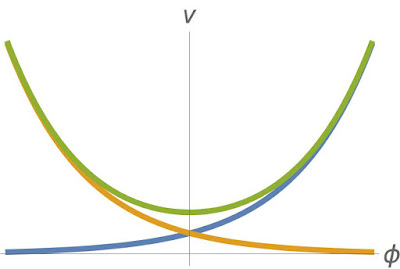## Friday, March 25, 2016

### How does loop quantum cosmology work?The evolution of the expectation value of the volume v of the universe is plotted. The blue and orange lines are the expanding and contracting branches in the Wheeler-de Witt theory. The green curve follows from loop quantum cosmology and exhibits a quantum bounce close to Planck density.

These days I have been working on lecture notes for an introductory lecture series on loop quantum gravity. An introductory article by Abhay Ashtekar introduced this subject via a discussion on loop quantum cosmology (LQC), where already many of the essential features of loop quantum gravity are present and can be studied in a simplified setting. I think that this is a very useful pedagogical approach and I also wanted to incorporate it into my lectures. I just finished my first draft of a lecture about this subject, focussing on a specific exactly soluble LQC model and its comparison to a similar quantisation using the Wheeler-de Witt framework. Mostly I follow the original paper, with some additional comments, slight rearrangements, and omission of more advanced material that is not necessary in an introductory course in my point of view. The current draft is available here, and comments are always welcome.

The short version goes as follows:

In both cases, Wheeler-de Witt and loop quantum cosmology, one quantises general relativity minimally coupled to a massless scalar field in a homogeneous and isotropic setting. At the classical level, one constructs a Dirac observable corresponding to the volume of the universe at a specific point in time specified by the value that the scalar field takes. As is well known, such models lead to either big bang or big crunch singularities.

One can now quantise using Wheeler-de Witt techniques (both the spatial volume and its conjugate momentum are well defined operators) and show that one can reduce the problem of finding the physical Hilbert space to that of solving a 1+1-dimensional Klein-Gordon equation due to a suitable choice of variables. On the physical Hilbert space, one can represent a Dirac observable corresponding to the volume at some specific value of the internal scalar field time $\phi$. One sees that the expectation value of these observables follows the classical trajectories, i.e. $V_0 e^{\pm \phi}$. A more in depth analysis based on consistent histories (not detailed in the lecture) also shows that superpositions of contracting and expanding branches do not lead to singularity resolution (see however this paper based on the Bohmian approach to quantum theory).

In loop quantum cosmology on the other hand one finds some small, but important differences in the computation. Due to the loop quantum gravity inspired Hilbert space, the spatial volume is a well defined operator, whereas its conjugate momentum $b$ exists only in exponentiated form $e^{i n b}$, $n \in \mathbb Z$. This reflects the underlying discrete quantum geometry. Therefore, one needs to regularise $b$ in terms of such U(1)-holonomies'', with the simplest choice being $\sin(b)$. Since we are working in Planck units, $b$, corresponding on-shell to the matter energy density, is small and $\sin(b)$ is an excellent approximation to $b$ if we are far away from the Planck density. At Planck density however, a departure from classical physics can be expected. Indeed, passage to the Klein-Gordon equation now requires a slightly different choice of variables, leading to a different form of the operator corresponding to the spatial volume on the physical Hilbert space. It turns out that the expectation value of this operator behaves as $V_{\text{min}} \cosh(\phi)$. The big bang / big crunch singularities are thus substituted by a quantum bounce.

#### 1 comment:

1.In his introductory article, Ashketar asks the question, "Can one construct a framework that cures the short-distance difficulties faced by the classical theory near singularities, while maintaining an agreement with it at large scales?”’
I have suggested that Einstein’s field equations are slightly wrong (because the equivalence principle is completely false for dark matter and even very slightly wrong for ordinary matter) — I have also suggested that Maxwell's equations are slightly wrong. By correcting Einstein's field equations and Maxwell's equations, most problems with singularities might be resolved.
Renormalization in quantum electrodynamics deals with infinite integrals that arise in perturbation theory. Such infinite integral might arise merely because Maxwell’s equations are incorrect at the Planck scale. Assume that leptons have structure at the Planck scale. I conjecture that, EVEN AFTER QUANTUM AVERAGING, Maxwell’s equations might be false at the Planck scale, because leptons have structure at the Planck scale. Let ρ represent the electric charge density (charge per unit volume). I conjecture that, in equation (19b) on page 23 of Einstein’s “The Meaning of Relativity” (5th edition), ρ should be replaced by the expression ρ/ (1 – (ρ^2 / (ρ(max))^2))^(1/2), where ρ(max) is the maximum of the absolute value of the electric charge density in the physical universe.
The preceding hypothesis makes the qualitative prediction that electromagnetic radiation emanating from sources near black holes might be unexpectedly large. M82 X-2 might be worth considering in this regard.
M82 X-2, en.wikipedia